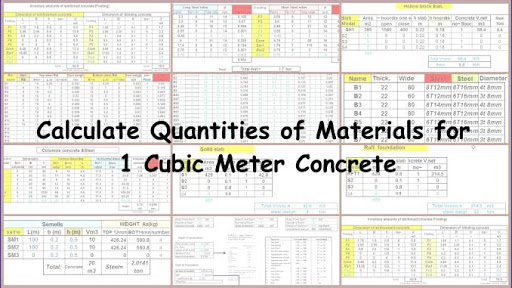# Calculate Quantities of Materials for Concrete Excel Sheet

Quantities of materials for the production of the required quantity of concrete of given mix proportions can be calculated by the absolute volume method. This method is based on the principle that the volume of fully compacted concrete is equal to the absolute volume of all the materials of concrete, i.e. cement, sand, coarse aggregates, and water. A Concrete structure may consist of beams, slabs, columns, and foundations, etc. based on the type of structure. The volume of concrete required for concrete structure can be calculated by summing up the volumes of each structural member or each part of members.Calculate Quantities of Materials for Concrete